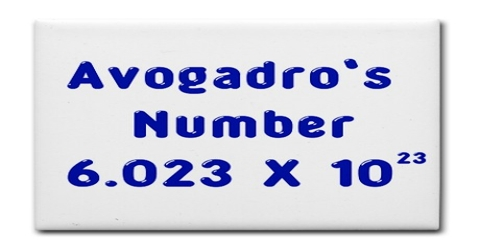ChemistryAccording to Avogadro’s hypothesis equal volumes of all gases under the same conditions or temperature and pressure contain the same number of molecules. This number, i.e., the number of molecules in 1 mole of a gas, is called Avogadro number. It is a fundamental constant of physical chemistry.

The number of atoms in 1 mole of atoms or the number of ions in 1 mole of ions has also the same value. The best value of this number is 6.023 x 1023.

Its value is, NA = 6.023 x 1028 mol-1

The basis for accepting this as fundamental constant in physical chemistry is that different types of accurate measurements based on different principles yield the same value. Some of the experiments which have established the value of the Avogadro number are given below;

(i) Distribution of very small solid particles in different lavers when these are suspended in a liquid medium

(ii) Microscopic observation of the random displacement of small particles. Both (i) and (ii) were carried out by Perrin with different particles and at various temperatures.

(iii) Determination of the charge of an electron by the oil-drop experiment of Millikan and the value of the Faraday constant of electrolysis.

(iv) From the determination of the charge on the alpha particles from radium by Rutherford.

(v) From a determination of the constants of radiation by Planck.

(vi) Direct determination of the wave-length of X-rays.

The usefulness and applications of the Avogadro number are numerous. For example, the mass of a single atom or molecule of a substance may be obtained by dividing the respective atomic or molecular mass by the Avogadro number. Thus, the mass of an atom of helium is 4.003/(6.023 x 1023) = 6.65 x10-24 g; the mass of a molecule of oxygen is 32.000 / (6.023 x 1023 = 5.32 x 10-23 g.

Avogadro number also enables one to calculate the number of molecules in a given volume of gas or a given mass of substance.